Previous: 3.3.2.1 Interatomic Screening Potential Up: 3.3.2 Nuclear Stopping Process Next: 3.3.3 Electronic Stopping Process

### 3.3.2.2 Average Nuclear Stopping Power

Although it is not relevant for the Monte-Carlo simulation of ion implantation it is quite interesting to make some statements of the average nuclear stopping power.

Knowing the interatomic potential it is possible to derive the average nuclear stopping power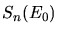which defines the average energy that is transfered by each nuclear collisions if the particle energy is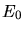.can be calculated by integrating the energy transfer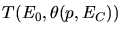of a nuclear collision event over all possible impact parameters.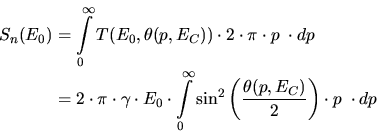(3.93)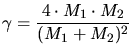(3.94)

Although there is no analytical solution for the average nuclear stopping power, a numerical solution can be derived using equations (3.90), (3.89) and (3.88) for the scattering angle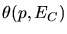. Therefore the so called dimensionless reduced energy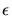and the dimensionless reduced nuclear stopping have to be introduced.

The reduced energy considers the dependence of the nuclear stopping power on the masses (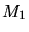and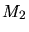) and the charges (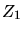and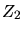) of the atoms involved in the scattering process. The relation between the real ion energyin units of keV and the reduced energy is given by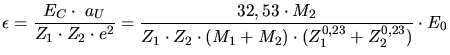(3.95)

This relation is also plotted in in Fig. 3.6 for various atom pairs relevant for semiconductor ion implantation to give an impression of the order of the reduced energy.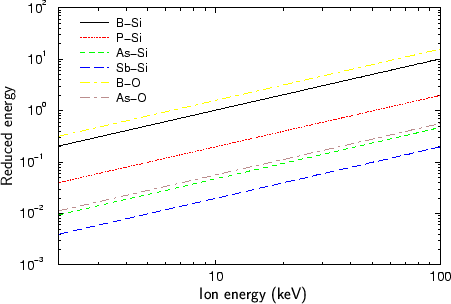The reduced nuclear stopping is defined by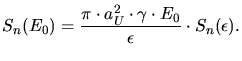(3.96)

It allows to find an analytical approximation to the numerical solution of the nuclear stopping power. The analytical approximation for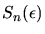is given by (3.97) and plotted in Fig. 3.7 .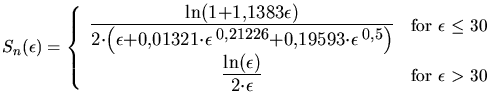(3.97)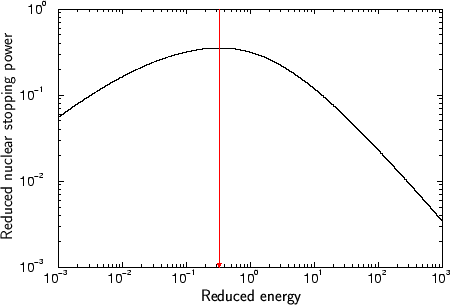Applying this approximation to (3.96) gives the nuclear stopping power as expressed in (3.98) in units of eV/(atom/cm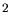), by additionally inserting (3.94), (3.95) and (3.92).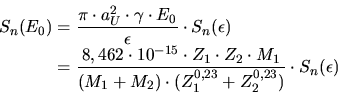(3.98)(3.97) has its maximum at a reduced energy of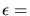0,327 where also the average nuclear stopping power has its maximum according to (3.98). The relating real particle energy where the average stopping has is maximum can be calculated by (3.95). Tab. 3.2 summarizes the approximate stopping power maxima for various atom pairs.

Table 3.2: Maxima of the average nuclear stopping power for various atom pairs.
 Incident particle Target particle Nuclear stopping power maximum (keV) Boron Silicon 3 Phosphorus Silicon 16 Arsenic Silicon 68 Antimony Silicon 160 Boron Oxygen 2 Phosphorus Oxygen 12 Arsenic Oxygen 58 Antimony Oxygen 143

A. Hoessiger: Simulation of Ion Implantation for ULSI Technology